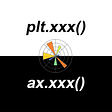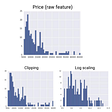# Confusion Matrix — Are you confused? (Part 1)

“Life is full of confusion. Confusion of love, passion, and romance. Confusion of family and friends. Confusion with life itself. What path we take, what turns we make. How we roll our dice.”

-Matthew Underwood

## Introduction

The confusion matrix is one of the most widely used evaluation metrics in the world of data science and machine learning. Although the mathematics operated behind this technique is easy, the terminologies often make it difficult to comprehend. It requires a long article to start at a slow pace and explain the concepts from scratch to the top. Here, I will write a series of articles to explain the working methodology of the confusion matrix. This article is written with a pre assumption that the reader is already aware of classification-based algorithms in machine learning.

## Definition

The confusion matrix is an evaluation metric often represented in the form of a matrix to evaluate the performance of a classification model.

Example

Consider that we are building a model based on any binary classification problem to predict whether an earthquake will happen or not.

Assume that our data consists of 1000 records, out of which we categorized 800 records as training data and the rest 200 records as testing data. After the training, we can test the performance of the trained model using our testing data which has 200 records.

Now, the question is how well our model has performed in an unknown data set (testing data). Most often, people go with metrics called “Accuracy” which calculates how much correct predictions were made out of the total number of required predictions by comparing it with a known output and this is undoubtedly a good way of evaluating the model. In our case, Let’s assume out of 200 records in the test data, our model predicted(classified) 160 records correctly. Hence the accuracy becomes 160/200 = 0.8 or we can consider this as 80% accuracy.

Good. Isn’t it?

But wait. Is this enough? Apart from this accuracy of 80%, there is much-hidden information that lies behind this accuracy and we need to decode this.

We have accuracy as,

Accuracy =

Total number of records predicted correctly /Total number of records allowed for testing

In other words, this is simply a percentage representation of correct predictions made by our model.

In our case, our model predicted that earthquakes will happen/will not happen accurately 160 times out of 200. It means that in the future when a real scenario demands us to predict whether an earthquake will happen or not, we can predict with an accuracy of 80%.

But still, we have no answer to the following questions if we think deeply,

· How many wrong predictions have more serious consequences?

· How many wrong predictions can be ignored without much diagnostics?

· Whether our correct predictions were truly useful in important scenarios?

· Out of all positive predictions (here, it means a prediction that conveys that the earthquake will happen), How much went to become correct?

· Out of all negative predictions (here, it means a prediction that conveys that the earthquake will not happen), How much went to become correct?

· Whether our model is better in positive prediction or negative prediction?

· Whether there is any positive/negative prediction that happened in a scenario that is very rare to happen in the future?

· Whether there is any positive/negative prediction that happened in a scenario that is very frequent to happen in the future?

· If there were multiple types of models such as Decision trees, KNN, Logistic regression, etc. Which was the best one?

A confusion matrix can answer a lot of questions like this and there is no doubt that these will give better insights into data. Hope now you have understood the importance of the confusion matrix. Let’s have a look at an example.

This is a typical matrix representation of the accuracy that we mentioned above. Now we aim to draw more conclusions from this matrix. This can be done by splitting this matrix more convincingly.

But before that let’s discuss the most 4 important terms present in a confusion matrix. The conceptual understanding of these 4 terms is a little bit confusing but it is important to understand this inference to diagnose a confusion matrix.

· True positive

· True negative

· False positive

· False negative

## True positive

We call the data point in the confusion matrix a true positive when we predicted a positive outcome and what happened is the same.

## True negative

We call the data point in the confusion matrix a true negative when we predicted a negative outcome and what happened is the same.

## False-positive

We call data points in the confusion matrix false positive when we predicted a positive outcome and what happened is a negative outcome.

This scenario is known as Type 1 error.

## False-negative

We call data points in the confusion matrix false negatives when we predicted a negative outcome and what happened is a positive outcome.

This scenario is known as Type 2 error.

“Often, Data scientists take many precautions so that a Type-2 error should not happen at any cost and it is considered as much dangerous as a Type-1 error.

Let’s imagine a situation in which we have predicted that an earthquake will happen but it didn’t happen. That’s okay, right?

We took some cautions that an earthquake will come but luckily it didn’t happen. So it’s like a boon in a bad prediction.

But what if we predicted that an earthquake will not happen and suddenly it got happened against our expectations?

We can’t even imagine that scenario for the loss we account for. That’s why Type-2 error is considered as more dangerous.”

Now what I mentioned above in definitions can be represented in a diagrammatic way as follows

Here,

Although accuracy is 80%, we are now able to infer that -

· There is a 5% chance that a worst-case prediction is possible to come from this model (Type 2 error).

· There is a 15% chance that a Type-I error is possible is to come from this model.

· This model is more inclined toward identifying a positive outcome rather than a negative outcome (Since True positive > True negative)

· True positives and True negatives constitute 80% of total data points in the confusion matrix which means that model can approximately achieve an 80% accuracy in its prediction when it is exposed to unknown data.

But these are not the advanced ones till now. There are 3 other evaluation metrics which are ratios derived from the above terms to get more conclusions. Those ratios are-

· Precision

· Recall

· F1 Score

We will discuss these ratio based metrics in part 2 of this article. Hope you got a good foundation in the understanding confusion matrix basics.

Confusion Matrix — Are you confused? (Part 2)

--

--

--

## More from Sanjay Nandakumar

Data scientist | ML Engineer | Statistician | https://www.quora.com/profile/Sanjay-Kumar-563 | https://www.linkedin.com/in/sanjay-nandakumar-8278229b/

Love podcasts or audiobooks? Learn on the go with our new app.

## Machine Learning Needs DataOps## This is better than A/B test## How to create a basic visualisation for a travel show’s journey iunTableau## Weight Decay and Its Peculiar Effects## plt.xxx(), or ax.xxx(), That Is The Question In Matplotlib## Hive Performance tuning## Converting Geographical Data in a Pandas Dataframe to a MongoDB Geopoint Object## Lessons in Public Transport Equity from the Majority World## Sanjay Nandakumar

Data scientist | ML Engineer | Statistician | https://www.quora.com/profile/Sanjay-Kumar-563 | https://www.linkedin.com/in/sanjay-nandakumar-8278229b/

## The Difference between Supervised and Unsupervised Learning## Different Normalization Techniques## Medical Insurance Charges Prediction — Linear Regression, SGD Regressor, Random Forest## K-Nearest Neighbors Classification